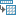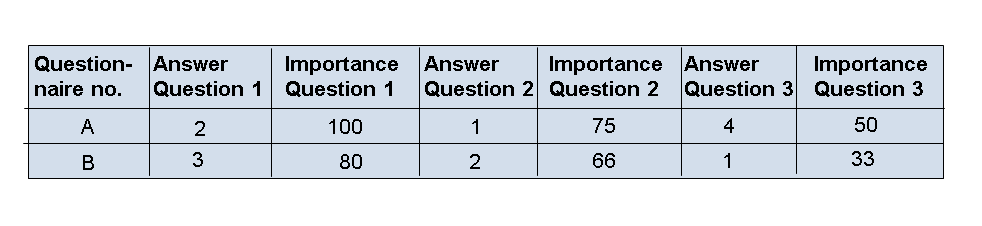Show TOC

# Transforming Data Records into ListsUse

Using transformation, you can transform repeat columns in a table into several rows. The system then generates several records in the output table for each individual data record in the input table.

This transformation is similar to transposition of a table. During transposition, rows and columns in the whole table are swapped. This transformation only transposes selected columns in the table however. All other columns are left unchanged.

Features

The type of input column should concur with the type of transposition field. The field content is copied using ABAP-MOVE logic and converted where necessary. If conversion is not possible, for example because the FLOAT value 1.00E30 is to be transferred into a decimal field of length 10, it causes the analysis process to terminate due to overflow.

Activities
1. On the Definition tab page, select the fields to transform. These are the fields to be filled by the transformation. In the example below, these are the columns Answer Question 1, Importance Question 1, Answer Question 2, Importance Question 2, Answer Question 3, and Importance Question 3. Characteristic Questionnaire is left unchanged. These columns are deleted from the output structure.

2. On the Transformation tab page, define the transformation field. The new column that adds the characteristic value to the column name is also defined here (with name, description and InfoObject for type). In the example below, this is the new column Question No..

3. Also define at least one transposition field on the Transformation tab page that is to be filled by the transformation. The fields defined here are inserted into the output structure of the node. In the example, these are the new columns Answer and Importance.

4. So far, you have described the new output structure. You now have to define the transformation rules that describe how the field contents are transferred from the old structure to the new one.

On the Details tab page, specify for each field in the original data record the row and column in the output table to insert the value. The row is specified with a characteristic value for the transformation field and the column through the selection of a transposition field.

Example

For column Importance Question 1, characteristic value 1 is entered as the characteristic value for Question No. while Importance is entered as the column for the new column.

Example

The answers to a questionnaire are available in table form. The table contains all of the answers to the questionnaire by line:For subsequent processing, this table should be put into a format where each data record contains the answer and importance of one question. When running the analysis process, a part of the column name (question 1/question 2/question 3) is converted into a characteristic value for a new column Question No. The repeating fields Answer and Importance are added to the new columns Answer and Importance: